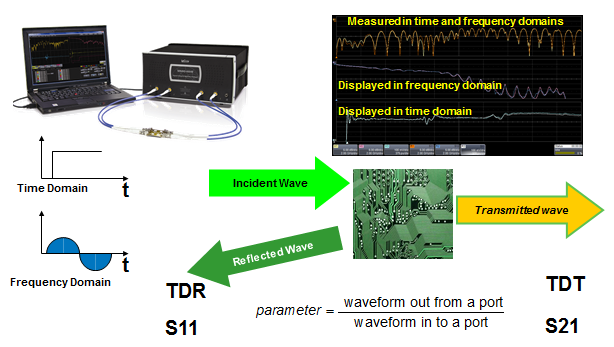## You need to test, we're here to help.

You need to test, we're here to help.

## 03 December 2014

### What S-Parameters Reveal About InterconnectsFigure 1: S-parameters are derived by applying an incident wave to an interconnect; we can consider this process in either the time or frequency domains
S-parameters are a popular means of characterizing an interconnect. By feeding the interconnect with a precision reference signal and measuring how much of that signal propagates through the connector and how much is reflected, we learn everything we need to know about its performance. This will be the first in a series of posts about the insights we can glean from S-parameters with practical examples of common measurement scenarios.

One way to derive the S-parameters of an interconnect is through the use of time-domain reflectometry. Basically, the idea is to send a fast-rising edge as the incident waveform to the DUT. Some portion of that waveform is reflected back to the input and is referred to as the time-domain reflected (TDR) wave. Meanwhile, some of the signal reaches the far end of the interconnection as the time-domain transmitted (TDT) wave (Figure 1).

It's in the frequency domain that we really get into S-parameters. That incident wave—which, for the sake of our example we'll call a sine wave—is composed of a series of sine waves, each with its own frequency, amplitude, and phase, that makes up that fast edge, which can be decomposed into its component parts. In the frequency domain, the TDR wave is called S11 or, sometimes, the return loss. The TDT wave is known as S21 or the insertion loss.Figure 2: S-parameters may be arrived at through direct measurement, simulation, or field solvers
Whether they're measured in the time or frequency domain, S-parameters are readily converted from one domain to the other. Using Fourier transforms, the time-domain response can be mathematically transformed into the frequency-domain response and back again with no loss or change of the data. Depending on what one is looking for, answers may be easier to come by in one or the other domain. For example, if we want to know the characteristic impedance of a uniform transmission line, a time-domain display of the response will get us an answer more quickly. But if we want to find the bandwidth of the transmission line, the frequency-domain display is more helpful.

S-parameters can be arrived at in a number of ways (Figure 2). They can be measured directly with an instrument such as Teledyne LeCroy's SPARQ signal integrity network analyzers, which can measure S-parameters from DC to 40 GHz and from two to 12 ports. SPARQ also automates all sorts of conversions. S-parameters can be simulated using circuit-simulation software such as SPICE. Then there's 2D and 3D electromagnetic field solvers. However, no matter how you approach it, the models contain the same information. Single-ended S-parameters can be converted into differential models. It's easier to measure single-ended elements and then mathematically convert them into differential elements.

In the previous paragraph we alluded to "ports," which are simply the terminations of the interconnect. Or, we could say that the port is the interface between the measurement and the interconnect; it's where we will connect to the measurement device. A port has two conductors, one being the signal path and one being the signal return or ground path. The port can be thought of as a tiny coaxial connector with its center signal pin and collar connected to ground.Figure 3: Measuring S11 from a one-port device

Let's consider a one-port device and take a look at a hypothetical measurement scenario. On the top left of Figure 3 is our network analyzer, which itself has a port in the form of its precision 50-Ω output connector. This port will be our signal source and also the input for the reflected signal from the DUT via a precision 50-Ω cable. If there's any variation from a 50-Ω impedance in the DUT, the analyzer will detect the resultant reflection.

With this kind of a one-port DUT, we can measure S11, which is the reflected wave divided by the incident wave. S11 has an associated magnitude and phase, so it will be described in terms of a complex number. In most cases, it's the magnitude that's the object of interest. Having said that, it's important to keep track of the phase of S11, which we'll need to know if we want to manipulate the S-parameters by addition, subtraction, or what have you.

In subsequent posts, we'll look at two-port S-parameters, as well as the connection between insertion loss and return loss. We'll also look at some important patterns we can look for in S11 and S21 and see what they tell us about our DUT.